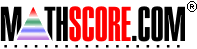Math Practice Online > free > lessons > Florida > 6th grade > Mean, Median, Mode

## Mean, Median, Mode

Calculate the mean, median, mode, and range of values.

 Sample Problems for Mean, Median, Mode Lesson for Mean, Median, Mode

### This topic aligns to the following state standards

Grade 2: Alg 1. Uses concrete objects, paper and pencil, or mental mathematics to solve real-world equations with one unknown (such as, There are 28 students in the room, and 16 brought their lunches. How many are buying lunch?).
Grade 3: Dat 2. Identifies the median and mode from a set of numerical data.
Grade 3: Dat 3. Identifies the range in a set of numerical data.
Grade 4: Dat 1. Identifies the mean, median and mode from a set of data.
Grade 5: Dat 2. Uses range and measures of central tendency in real-world situations.
Grade 6: Dat 2. Finds the range, mean, median, and mode of a set of data.
Grade 7: Dat 1. Finds the range, mean, median, and mode of data from a table, chart, or graph.
Grade 8: Dat 1. Finds the mean, median, and mode of a set of data using raw data, tables, charts, or graphs.

Copyright Accurate Learning Systems Corporation 2008.